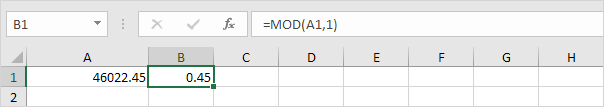# MOD

The MOD function in Excel gives the remainder of a division. You can use the MOD (Modulus) function to determine whether a number is divisible by another number or to check if a number is even or odd.

### MOD examples

1. The MOD function below returns 9.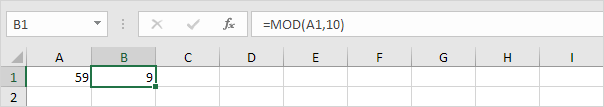Explanation: 59 divided by 10 equals 5 with a remainder of 9.

2. The MOD function below returns 1.Explanation: 7 divided by 2 equals 3 with a remainder of 1.

3. The MOD function below returns 0.Explanation: 36 divided by 6 equals 6 with a remainder of 0.

### Combine MOD with other Functions

Let's explore the power of the MOD function by combining it with other Excel functions!

1. Use IF and MOD to determine if a number is divisible by another number. For example, check if the number in cell A1 is divisible by 8.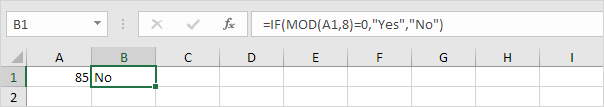Explanation: 85 divided by 8 equals 10 with a remainder of 5. The MOD function returns 5. As a result, the IF function returns No.

2. Change the value in cell A1 to 88.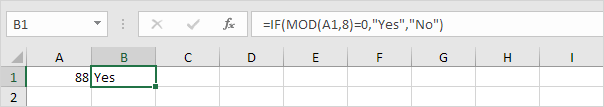Explanation: 88 divided by 8 equals 11 with a remainder of 0. The MOD function returns 0. As a result, the IF function returns Yes (88 is divisible by 8).

You can also use IF and MOD to check if a number is even or odd.

3. Even numbers divided by 2 always give a remainder of 0. For example, 28 divided by 2 equals 14 with a remainder of 0. As a result, the formula below returns Even.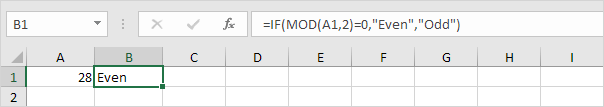4. Odd numbers divided by 2 always give a remainder of 1. For example, 29 divided by 2 equals 14 with a remainder of 1. As a result, the formula below returns Odd.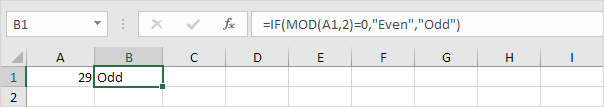Let's check out another cool example.

5. The formula below returns 0 (see orange arrows) for every 3rd row.Explanation: the ROW function returns the row number of a cell. For the first row, MOD(1,3) = 1 because 1 divided by 3 equals 0 with a remainder 1. For the third row, MOD(3,3) = 0 because 3 divided by 3 equals 1 with a remainder of 0.

6. Use this formula to sum every 3rd row in Excel.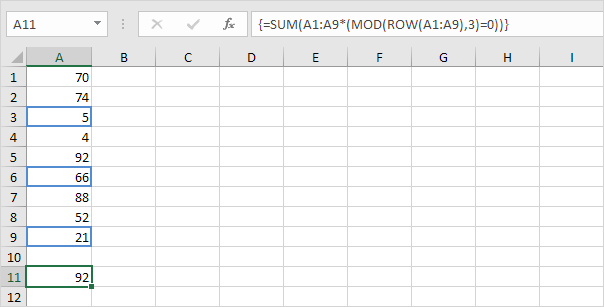### Extract Fractional Part

To extract the fractional part of a number, use the MOD function with a divisor (second argument) of 1.

1. The MOD function below returns the fractional part of the value in cell A1.Explanation: 3 divided by 1 equals 3 with a remainder of 0.45.

2. Knowing this, we can use the MOD function in Excel to extract the time from a datetime value.Explanation: dates are stored as numbers in Excel and count the number of days since January 0, 1900. The fractional part represents the time as a fraction of a day.

3. To clearly see this, select the range A1:B1 and change the number format to General. The result is a simple modulo operation.